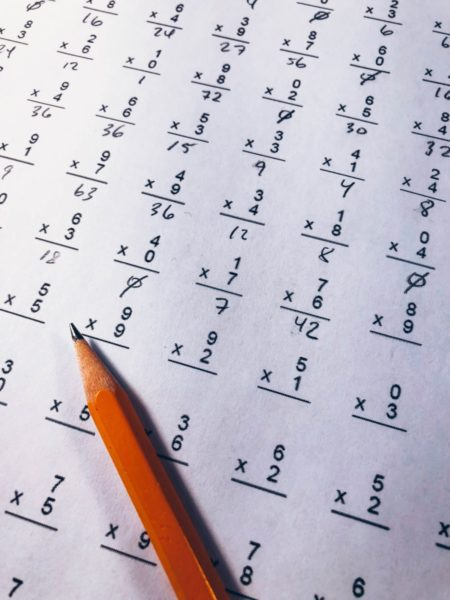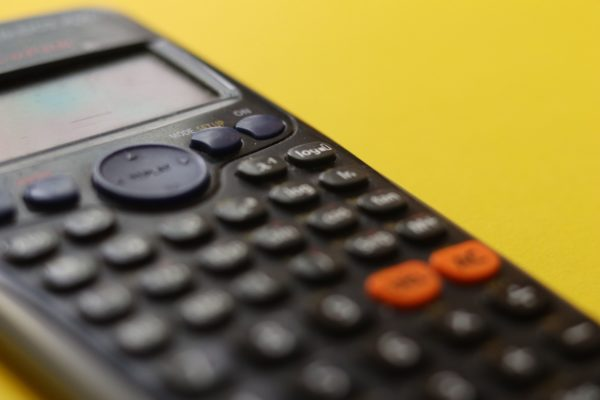Latest News

## Maths

Our Mathematics curriculum follows a mastery approach that aims for deep and sustainable learning; learning that is rooted in an appreciation of the connectedness of mathematical ideas and based on an understanding of the underlying structures. It emphasises the need to go beyond being able to memorise facts and practise procedures and routines whilst also focusing on the three aims of the National Curriculum: fluency, reasoning and problem solving. Students are encouraged to develop confidence in and a positive attitude towards mathematics and to recognise the importance of mathematics in their own lives and to society. They will also build a strong mathematical foundation for future studies at higher level post-16. The GCSE course in mathematics enables students to acquire, select and apply mathematical techniques to solve problems; reason mathematically, make deductions and inferences and draw conclusions; comprehend, interpret and communicate mathematical information in a variety of forms appropriate to the information and context.

“focusing on the three aims of the National Curriculum: fluency, reasoning and problem solving.”

Throughout their time with us, students will also be given the opportunity to engage with various numeracy days and mathematical competitions such as the National Young Mathematicians’ Awards and the UK Mathematics Trust’s Maths Challenges.

###Year 7

Number is at the heart of our Year 7 curriculum as this fundamental strand underpins all other areas of their mathematical journey. In Year 7, the curriculum is strongly linked to the KS2 curriculum to enable students to build on their prior learning and connect this to new concepts. Calculator use is introduced in Year 7 to further develop number sense. Algebra is used to generalise whenever possible as is introduced early to enable this.  Students will spend longer periods of time studying each unit of work to ensure a robust and embedded understanding. Higher attaining students are challenged through depth rather than acceleration onto new content.

### Year 8

In Year 8 students build on their knowledge and skills gained from Year 7 and KS2 through learning about how to use bar models to understand proportional relationships. Many more algebraic concepts are introduced to enable links to be established between geometry and algebraic manipulation. Calculator use is encouraged in Year 8 alongside regular opportunities for students to practise their mental Maths when appropriate. Students in Year 8 are also introduced to statistical measures and probability.

### Year 9

In Year 9, students are introduced to additional algebraic manipulation, working with polynomials. Following this, students continue their study of coordinate geometry, leading to the application of both in the study of quadratic graphs. Students also spend the time working with more advanced 2D

and 3D geometric problems, with focus on angles, construction and congruence before the introduction of Pythagoras’ Theorem and the application of surds. Later, students build on the study of probability in Year 8, with the introduction of Venn Diagrams and Frequency Trees.

###During Year 10 students build on prior learning from subsequent years to learn about Trigonometry and its applications. Additional algebraic techniques are introduced to provide students with more tools for solving complex problems. Links between circles and triangles are investigated and applied to further coordinate geometry problems. Statistical representations are explored with opportunities for students to analyse data in a variety of ways.

### Year 11

Tier decisions are made in Year 11 to ensure that students are thoroughly prepared for their GCSE examination in Mathematics. Higher tier students deepen their existing knowledge and skills by exploring further similarity and congruence, vector geometry and loci problems. Foundation students will have an opportunity to further strength and deepen their understanding and knowledge of the most challenging GCSE concepts including working with quadratics and percentage problems. Following mock examinations, bespoke plans are created to suit the needs of individual classes.

### Further Mathematics

Students in Year 10 and 11 are given the opportunity to study Further Mathematics to help the transition of students to Mathematics beyond Year 11. AQA Level 2 Certificate in Further Maths is a unique qualification designed to stretch and challenge high achieving mathematicians who either already have, or are expected to achieve the top grades in GCSE Mathematics or are likely to progress to study A-level Mathematics and possibly Further Mathematics. High-achieving students are introduced to AS topics that will help them develop skills in algebra, geometry, calculus, matrices, trigonometry, functions and graphs.

### Homework

Homework is an important part of the course and will be set once a week. The nature of the tasks set will vary and may include the follow up of class-work activities, computer set tasks, combination of electronic homework and paper-based homework are used.

“Using mathematics, you see the world beyond your imagination”